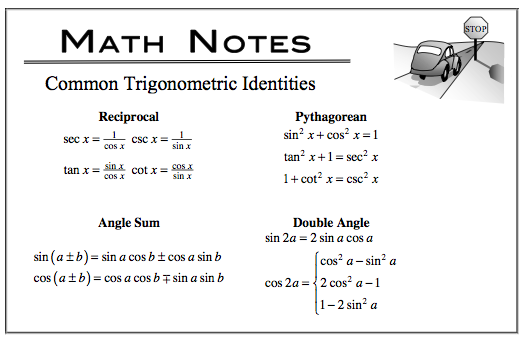### Home > APCALC > Chapter 5 > Lesson 5.3.3 > Problem5-135

5-135.

If $y = (\operatorname{cos}(x) + \operatorname{sin}(x))^2$, find $\frac { d y } { d x }$ using two different methods. Then demonstrate that the two results are equal. Homework Help ✎Method 1: Before you differentiate, expand and simplify using trig identities. Then use the Product Rule to differentiate.
Method 2: Chain Rule.

The results might not look the same. This is where you get to practice proving a trig identity (in other words, proving that the two results are equivalent.)